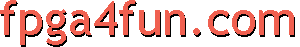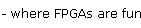## PWM DAC 1 - PWM (Pulse Width Modulation)

A PWM takes an input value of any width and creates an output that is just one-bit wide.

#### PWM using a free-running counter

That's the simplest PWM we can make.

```module PWM(
input clk,
input [3:0] PWM_in,
output PWM_out
);

reg [3:0] cnt;
always @(posedge clk) cnt <= cnt + 1'b1;  // free-running counter

assign PWM_out = (PWM_in > cnt);  // comparator
endmodule
```

We choose a 4bit PWM here so the PWM period is 16. The input can go from 0 to 15 so the PWM output ratio goes from 0% to 15/16=93%. If you need to be able to go up to 100%, the input needs to have an extra bit.

The code works fine, although it is a bit naive in its current form because the input must be fixed (or change only when the counter overflows = goes back to 0). Otherwise the output will glitch. So most likely a bit of extra logic is required (usually in the form of a latch capturing the input at the right time).

#### PWM using a loadable up-down counter

That's a slightly more sophisticated design.

```module PWM(
input clk,
input [3:0] PWM_in,
output PWM_out
);

reg [3:0] cnt;
reg cnt_dir;  // 0 to count up, 1 to count down
wire [3:0] cnt_next = cnt_dir ? cnt-1'b1 : cnt+1'b1;
wire cnt_end = cnt_dir ? cnt==4'b0000 : cnt==4'b1111;

always @(posedge clk) cnt <= cnt_end ? PWM_in : cnt_next;
always @(posedge clk) cnt_dir <= cnt_dir ^ cnt_end;
assign PWM_out = cnt_dir;
endmodule
```

It is using a loadable up-down counter, and doesn't need the output comparator. Interestingly, it is not quite equivalent to the first design as the output period has 17 states instead of 16 (output goes from 1/17=6% to 16/17=94%).